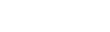# In this article, we will discuss how an RNN can be trained to map an input sequence to an output sequence that is not essentially of the same length.Mansoor Ahmed

3 months ago | 2 min read
Follow### Introduction

Encoder-Decoder Sequence-to-Sequence Models are famous for diverse tasks. These models are a distinctive class of Recurrent Neural Network architectures. We often use them to solve complex Language problems. For example;

• Machine translation
• Video captioning
• Image captioning
• Creating Chatbots
• Text Summarization

In this article, we will discuss how an RNN can be trained to map an input sequence to an output sequence that is not essentially of the same length.

### Description

The key idea behind the architecture of this model is to allow it to process input where we do not constrain the length.

• One RNN would be used as an encoder, and another as a decoder.
• The output vector made by the encoder and the input vector provided to the decoder will take a fixed size.
• Though, they do require not them to be equal.
• The output made by the encoder may either be given as a whole chunk.
• Also, it can be related to the hidden units of the decoder unit at every time step.

### How the Encoder-Decoder Sequence to Sequence Model works?

We will go over the following example in order to completely know the model’s fundamental logic:

### Encoder

• This is a stack of many recurrent units. LSTM or GRU cells for good performance.
• Each accepts a single element of the input sequence.
• It gathers information for that element and spreads it forward.
• An input sequence is a group of all words from the question in the question-answering problem.
• Every word is denoted as x_i where i is the order of that word.
• The hidden states h_i are calculated using the formula:\

### Encoder Vector

• Encoder Vector is the last hidden state.
• It is produced from the encoder part of the model.
• It is computed using the formula above.
• This vector objects to summarize the information for all input elements to support the decoder make correct predictions.
• It performs as the first hidden state of the decoder part of the model.

### Decoder

• As we can realize, we are just using the preceding hidden state to compute the next one.
• The output y_t at time step t is calculated using the below formula:

UpvoteCreated by

Mansoor Ahmed

Follow

Technologies in industry 4.0

Chemical Engineer, web developer and Tech writerPost

Upvote

Downvote

Comment

Bookmark

Share

Related Articles